# How To Find Z Parameters of Two Port Network (Examples)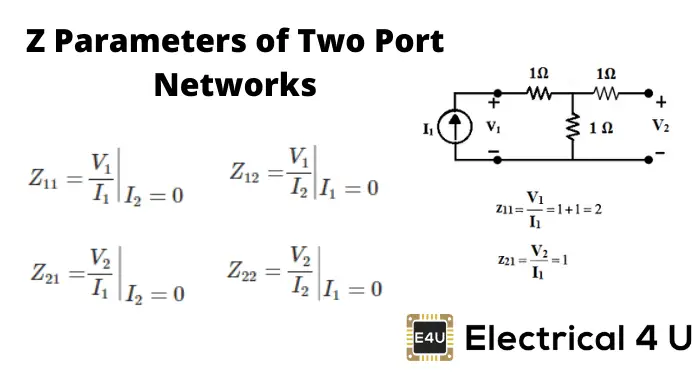## What are Z Parameters?

Z parameters (also known as impedance parameters or open-circuit parameters) are properties used in electrical engineering to describe the electrical behavior of linear electrical networks. These Z-parameters are used in Z-matrixes (impedance matrixes) to calculate the incoming and outgoing voltages and currents of a network.

Z-parameters are also known as “open-circuit impedance parameters”, as they are calculated under open-circuit conditions. That is to say that Ix=0, where x=1, 2 refers to the input and output currents flowing through the ports of a two port network.

Z parameters are commonly used alongside Y parameters, h parameters, and ABCD parameters to model and analyze transmission lines.

## How to Find Z Parameters in Two Port Networks

The below example goes over how to calculate the Z parameters of a two-port network. Note that Z parameters are also known as impedance parameters, and these terms are used interchangeably in these examples.

The input and output of a two port network can be either voltage or current.

If the network is voltage driven, that can be represented as below.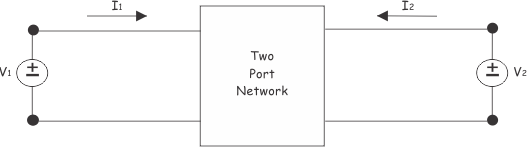If the network is driven by current, that can be represented as shown below.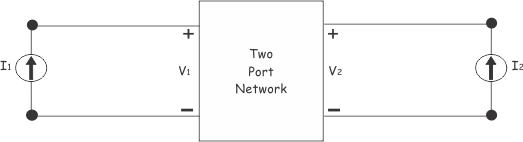From, both of the figures above, it is clear that there are only four variables. One pair of voltage variables V1 and V2 and one pair of current variables I1 and I2. Thus, there are only four ratios of voltage to current, and those are,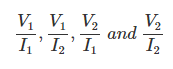These four ratios are considered as parameters of the network. We all know,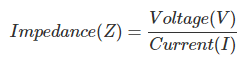This is why these parameters are called either impedance parameter or Z parameter.
The values of these Z parameters of a two port network, can be evaluated by making once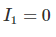and another once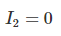Let us explain in brief. For that, first, we make the output port of the network open circuited as shown below.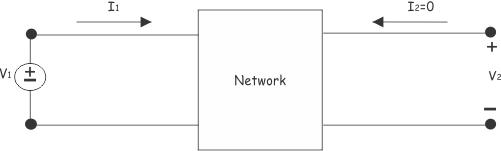In this case, as the output is open, there will be no current in the output port. i.e.In this condition, the ratio of input voltage to input current is mathematically represented as,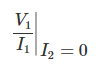This known as the input impedance of the network, while the output port is open. This is denoted by Z11
So, finally,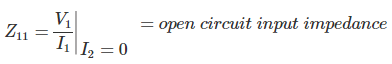Similarly,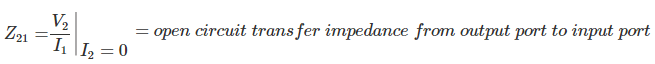Now, Voltage source V2 is connected across port 2 that is the output port, and the port 1 or input port is kept open as shown below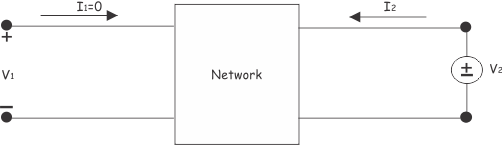Now, the ratio of V2 and I2 at I1=0 is,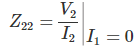This is called open circuit output impedance. Similarly,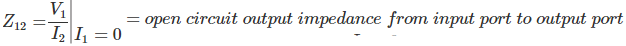Thus,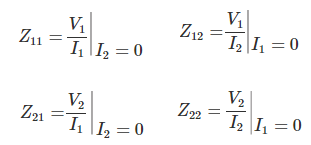Since all these above-shown Z parameters have been obtained by open circuiting output port or input port, the parameters are also referred to as open circuit impedance parameters.

Now, we can relate all voltage and current variables of a two port network by these Z parameters.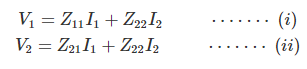These two equations can be represented in matrix form, as shown below,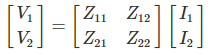In the equation (i), if we put I2 = 0, We get,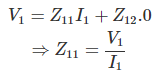Similarly, if we put I1=0, in the same equation, we get,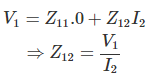In the same way, by putting I2 = 0 and I1 = 0 alternatively in equation (ii) We can prove,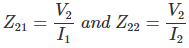Z11 and Z22 are also referred to as driving point impedance.
Z21 and Z12 are also referred to as transfer impedance. For better understanding, let us take the circuit below,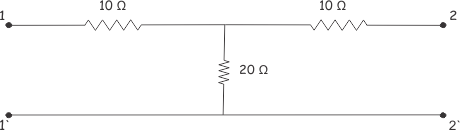Let us put a voltage source V1 at the input,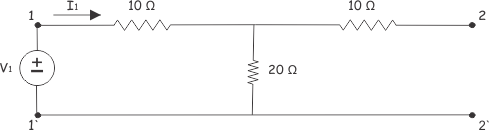Now,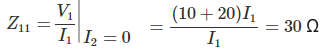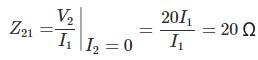Now, let us connect one voltage source V2 at the output port and leave the input port as open as shown, below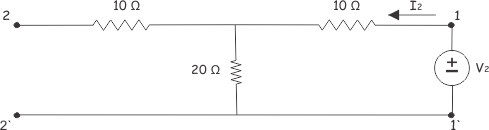Now,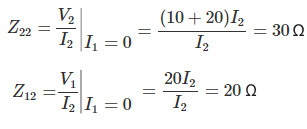So, Here,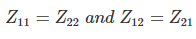When in a two port network, we get,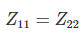We can call it a symmetrical network. Since, here,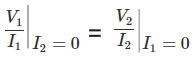As this ratio is the same, the same voltage at any of the port results in the same currents in the network.

That means if we apply voltage V1 at the output port then the output current will be I1. That means the network will have a mirror-like symmetry between output and input ports, in respect of the imaginary central line.
When we get,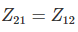Means,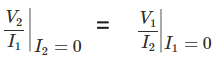That means, if input excitation and output response of the network are interchanged, the transfer impedance remains the same.

Suppose, V is the input voltage and I is the output current in the network as shown below.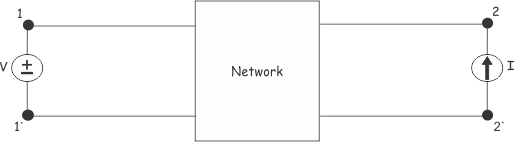Now if we connect a current source of I at the input port, so the voltage response of the network would be, V, at the output port.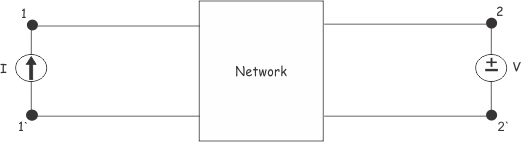This is because the ratio of voltage to current between input and output remains the same in both conditions. This is Reciprocity Theorem. The two port network behave like that is referred to as a reciprocal network.

For a symmetrical network,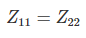For reciprocal network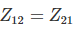Want To Learn Faster? 🎓
Get electrical articles delivered to your inbox every week.
No credit card required—it’s 100% free.